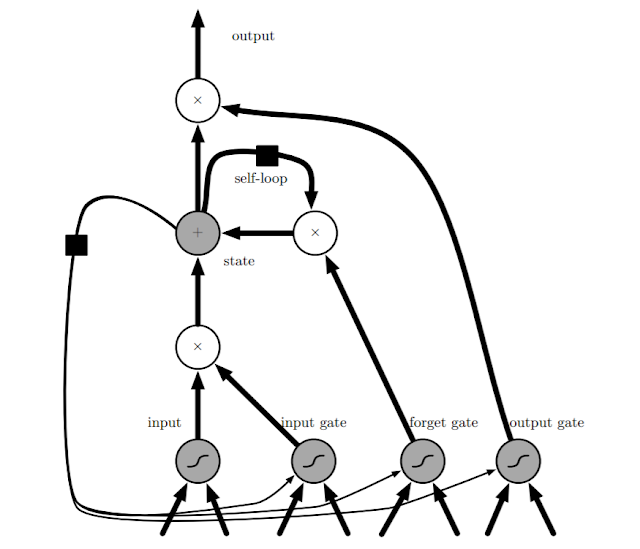## Thursday, September 26, 2019

### Why attention?

#### Resource saving

Only need sensors where relevant bits are
(e.g. fovea vs. peripheral vision)
• Only compute relevant bits of information
(e.g. fovea has many more ‘pixels’ than periphery)

#### Variable state manipulation

• Manipulate environment (for all grains do: eat)
• Learn modular subroutines (not state)

### Some forms of attention that you might not notice:

•  Pooling
• Iterative Pooling

Ref:

## Monday, September 23, 2019

### Mutual Information

Mutual Information
$$I(X ; Y)=D_{\mathrm{KL}}\left(P_{(X, Y)} \| P_{X} \otimes P_{Y}\right)$$

Intuitively, mutual information measures the information that $X$ and $Y$ share: It measures how much knowing one of these variables reduces uncertainty about the other.

\begin{aligned} \mathrm{I}(X ; Y) & \equiv \mathrm{H}(X)-\mathrm{H}(X | Y) \\ & \equiv \mathrm{H}(Y)-\mathrm{H}(Y | X) \\ & \equiv \mathrm{H}(X)+\mathrm{H}(Y)-\mathrm{H}(X, Y) \\ & \equiv \mathrm{H}(X, Y)-\mathrm{H}(X | Y)-\mathrm{H}(Y | X) \end{aligned}

where $H(Y|X)$ means Conditional Entropy, defined as:

$$\mathrm{H}(Y | X)=-\sum_{x \in \mathcal{X}, y \in \mathcal{Y}} p(x, y) \log \frac{p(x, y)}{p(x)}$$

\begin{aligned} \mathrm{H}(Y | X) & \equiv \sum_{x \in \mathcal{X}} p(x) \mathrm{H}(Y | X=x) \\ &=-\sum_{x \in \mathcal{X}} p(x) \sum_{y \in \mathcal{Y}} p(y | x) \log p(y | x) \\ &=-\sum_{x \in \mathcal{X}} \sum_{y \in \mathcal{Y}} p(x, y) \log p(y | x) \\ &=-\sum_{x \in \mathcal{X}, y \in \mathcal{Y}} p(x, y) \log p(y | x) \\ &=-\sum_{x \in \mathcal{X}, y \in \mathcal{Y}} p(x, y) \log \frac{p(x, y)}{p(x)} \\ &=\sum_{x \in \mathcal{X}, y \in \mathcal{Y}} p(x, y) \log \frac{p(x)}{p(x, y)} \end{aligned}

## Monday, September 16, 2019

### RNN

Assume the RNN is with the following architecture, with hyperbolic tangent activation function, output is discrete.

From time $t=1$ to $t=\tau$ , we apply the update equations:
\begin{aligned} \boldsymbol{a}^{(t)} &=\boldsymbol{b}+\boldsymbol{W} \boldsymbol{h}^{(t-1)}+\boldsymbol{U} \boldsymbol{x}^{(t)} \\ \boldsymbol{h}^{(t)} &=\tanh \left(\boldsymbol{a}^{(t)}\right) \\ \boldsymbol{o}^{(t)} &=\boldsymbol{c}+\boldsymbol{V} \boldsymbol{h}^{(t)} \\ \hat{\boldsymbol{y}}^{(t)} &=\operatorname{softmax}\left(\boldsymbol{o}^{(t)}\right) \end{aligned}

The total loss for a given sequence of $x$ values paired with a sequence of $y$ values would then be just the sum of the losses over all the time steps. If $L^{(t)}$  is the negative log-likelihood of $y^{(t)}$ given $x^{(1)}, x^{(2)}, ..., x^{(t)}$, then

\begin{aligned} & L\left(\left\{\boldsymbol{x}^{(1)}, \ldots, \boldsymbol{x}^{(\tau)}\right\},\left\{\boldsymbol{y}^{(1)}, \ldots, \boldsymbol{y}^{(\tau)}\right\}\right) \\=& \sum_{t} L^{(t)} \\=&-\sum_{t} \log p_{\text {model }}\left(y^{(t)} |\left\{\boldsymbol{x}^{(1)}, \ldots, \boldsymbol{x}^{(t)}\right\}\right) \end{aligned}

For each node $\boldsymbol{N}$:

$$\frac{\partial L}{\partial L^{(t)}}=1$$

### LSTMLSTM architecture from Deep Learning book

Forget gate:   $$f_{i}^{(t)}=\sigma\left(b_{i}^{f}+\sum_{j} U_{i, j}^{f} x_{j}^{(t)}+\sum_{j} W_{i, j}^{f} h_{j}^{(t-1)}\right)$$

where $\sigma$ is the sigmoid function

Input gate:
$$g_{i}^{(t)}=\sigma\left(b_{i}^{g}+\sum_{j} U_{i, j}^{g} x_{j}^{(t)}+\sum_{j} W_{i, j}^{g} h_{j}^{(t-1)}\right)$$

Output gate:
$$q_{i}^{(t)}=\sigma\left(b_{i}^{o}+\sum_{j} U_{i, j}^{o} x_{j}^{(t)}+\sum_{j} W_{i, j}^{o} h_{j}^{(t-1)}\right)$$

LSTM Cell Internal State:
$$s_{i}^{(t)}=f_{i}^{(t)} s_{i}^{(t-1)}+g_{i}^{(t)} \sigma\left(b_{i}+\sum_{j} U_{i, j} x_{j}^{(t)}+\sum_{j} W_{i, j} h_{j}^{(t-1)}\right)$$

Output(hidden state):
$$h_{i}^{(t)}=\tanh \left(s_{i}^{(t)}\right) q_{i}^{(t)}$$

Ref:

1. Goodfellow, I., Bengio, Y., & Courville, A. (2016). Deep learning. MIT press.